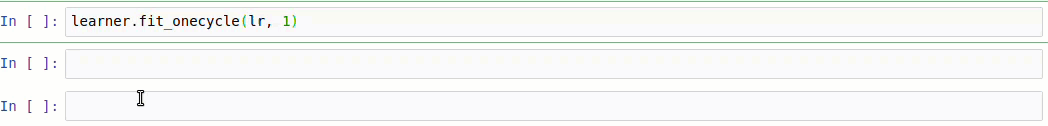TorchFit is a simple, easy-to-use, and minimalistic training-helper for PyTorch

# TorchFit

TorchFit is a bare-bones, minimalistic training-helper for PyTorch that exposes an easy-to-use fit method in the style of fastai and Keras.

TorchFit is intended to be minimally-invasive with a tiny footprint and as little bloat as possible. It is well-suited to those that are new to training models in PyTorch.

## Usage

# normal PyTorch stuff
model = create_your_pytorch_model()

# wrap model and data in Learner
import torchfit

# estimate LR using Learning Rate Finder
learner.find_lr()

# train using 1cycle learning rate policy
learner.fit_onecycle(1e-4, 3)

# plot training vs. validation loss
learner.plot('loss')

# make predictions as easy as in Keras

# save model and reload later
learner.save('/tmp/mymodel')


#### TorchFit Training Loop## Features

#### Learning Rate Finder

learner.find_lr()

#### A fit method for Training

# Examples
learner.fit(lr, epochs)
learner.fit_onecycle(lr, epochs)
learner.fit(lr, epochs, schedulers=[scheduler])


#### Easy-to-Execute Testing and Predictions

# Examples

text = 'Shares of IBM rose today.'
predicted_label = learner.predict_example(text, preproc_fn=preprocess, labels=labels)


learner.fit_onecycle(lr, 1, accumulation_steps=8)

learner.fit_onecycle(lr, 1, gradient_clip_val=1)

#### Mixed Precision Training

torchfit.Learner(model, train_loader, val_loader=val_loader, use_amp=True, amp_level='O2')

#### Multi-GPU Training and GPU Selection

To train on first two GPUs (0 and 1):

learner = torchfit.Learner(model, train_loader, val_loader=test_loader, gpus=[0,1])

To train only on the second GPU, one can do either this:

learner = torchfit.Learner(model, train_loader, val_loader=test_loader, gpus=)

or this...

learner = torchfit.Learner(model, train_loader, val_loader=test_loader, device='cuda:1')

#### Resetting Weights of Model

learner.reset_weights()

learner.save('/tmp/mymodel')


## Installation

After ensuring PyTorch is installed, install TorchFit with:

pip3 install torchfit



## Project details

This version0.2.50.2.40.2.30.2.20.2.10.2.00.1.0

Uploaded source